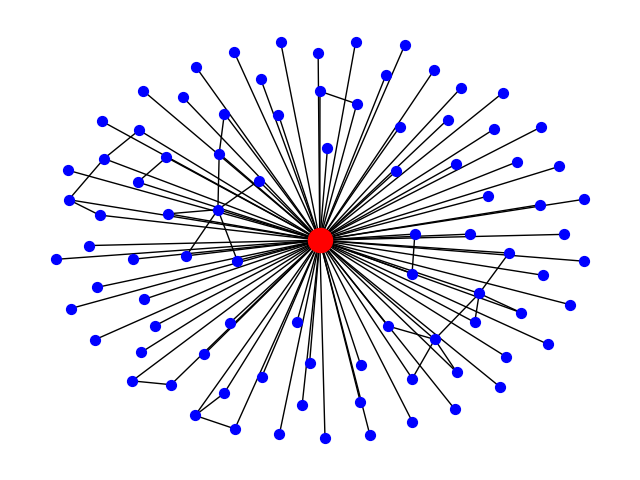# Ego Graph#

Example using the NetworkX ego_graph() function to return the main egonet of the largest hub in a Barabási-Albert network.```from operator import itemgetter

import matplotlib.pyplot as plt
import networkx as nx

# Create a BA model graph - use seed for reproducibility
n = 1000
m = 2
seed = 20532
G = nx.barabasi_albert_graph(n, m, seed=seed)

# find node with largest degree
node_and_degree = G.degree()
(largest_hub, degree) = sorted(node_and_degree, key=itemgetter(1))[-1]

# Create ego graph of main hub
hub_ego = nx.ego_graph(G, largest_hub)

# Draw graph
pos = nx.spring_layout(hub_ego, seed=seed)  # Seed layout for reproducibility
nx.draw(hub_ego, pos, node_color="b", node_size=50, with_labels=False)

# Draw ego as large and red
options = {"node_size": 300, "node_color": "r"}
nx.draw_networkx_nodes(hub_ego, pos, nodelist=[largest_hub], **options)
plt.show()
```

Total running time of the script: ( 0 minutes 0.081 seconds)

Gallery generated by Sphinx-Gallery